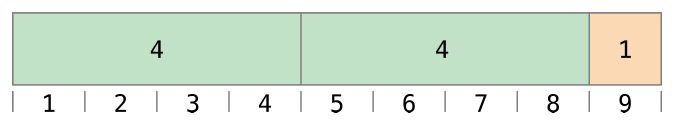# Swift学习之路-基本运算符

## 前言

Swift 在支持 C 中的大多数标准运算符的同时也增加了一些排除常见代码错误的能力。赋值符号（ `=` ）不会返回值，以防它被误用于等于符号（ `==` ）的意图上。算数符号（ `+ , - , * , / , %` 以及其他）可以检测并阻止值溢出，以避免你在操作比储存类型允许的范围更大或者更小的数字时得到各种奇奇怪怪的结果。如同 溢出操作符 中描述的那样，你可以通过使用 Swift 的溢出操作符来选择进入值溢出行为模式。

Swift 提供了两种 C 中没有的区间运算符（ `a..<b``a...b` ），来让你便捷表达某个范围的值。

## 专门用语

• 一元运算符对一个目标进行操作（比如 `-a` ）。一元前缀运算符在目标之前直接添加（比如`!b`），同时一元后缀运算符直接在目标末尾添加（比如`c!` ）。
• 二元运算符对两个目标进行操作（比如 `2 + 3` ）同时因为它们出现在两个目标之间，所以是中缀。
• 三元运算符操作三个目标。如同 C，Swift语言也仅有一个三元运算符，三元条件运算符（ `a ? b : c` ）。

## 赋值运算符

``````let b = 10
var a = 5
a = b
// a 的值现在是 10``````

``````let (x, y) = (1, 2)
// x 等于 1, 同时 y 等于 2``````

``````if x = y {
// 这是不合法的, 因为 x = y 并不会返回任何值。
}``````

## 算术运算符

Swift 对所有的数字类型支持四种标准算术运算符：

• 加 ( + )
• 减 ( - )
• 乘 ( * )
• 除 ( / )
``````1 + 2 // equals 3
5 - 3 // equals 2
2 * 3 // equals 6
10.0 / 2.5 // equals 4.0``````

``"hello, " + "world" // equals "hello, world"``

### 余数运算符``9 % 4 // equals 1``

`a = (b x some multiplier) + remainder`

`9``4` 插入到等式当中去：

`9 = (4 x 2) + 1`

`a` 是负数时也使用相同的方法来进行计算：

``-9 % 4 // equals -1``

`-9``4` 插入到等式当中：

`-9 = (4 x -2) + -1`

`b` 为负数时它的正负号被忽略掉了。这意味着 `a % b``a % -b` 能够获得相同的答案。

### 一元减号运算符

``````let three = 3
let minusThree = -three // minusThree equals -3
let plusThree = -minusThree // plusThree equals 3, or "minus minus three"``````

### 一元加号运算符

``````let minusSix = -6
let alsoMinusSix = +minusSix // alsoMinusSix equals -6``````

## 组合赋值符号

``````var a = 1
a += 2
// a is now equal to 3``````

## 比较运算符

Swift 支持所有 C 的标准比较运算符：

• 相等 ( `a == b` )
• 不相等 ( `a != b` )
• 大于 ( `a > b` )
• 小于 ( `a < b` )
• 大于等于 ( `a >= b` )
• 小于等于 ( `a <= b` )

Swift 同时也提供两个等价运算符（ ===  和 !== ），你可以使用它们来判断两个对象的引用是否相同。参考 类和结构体 章节来了解更多。

``````1 == 1 // true, because 1 is equal to 1
2 != 1 // true, because 2 is not equal to 1
2 > 1 // true, because 2 is greater than 1
1 < 2 // true, because 1 is less than 2
1 >= 1 // true, because 1 is greater than or equal to 1
2 <= 1 // false, because 2 is not less than or equal to 1``````

``````let name = "world"
if name == "world" {
print("hello, world")
} else {
print("I'm sorry \(name), but I don't recognize you")
}
// prints "hello, world", because name is indeed equal to "world"``````

``````(1, "zebra") < (2, "apple")   // true because 1 is less than 2
(3, "apple") < (3, "bird")    // true because 3 is equal to 3, and "apple" is less than "bird"
(4, "dog") == (4, "dog")      // true because 4 is equal to 4, and "dog" is equal to "dog"``````

Swift 标准库包含的元组比较运算符仅支持小于七个元素的元组。要比较拥有七个或者更多元素的元组，你必须自己实现比较运算符。

## 三元条件运算符

``````if question {
} else {
}``````

``````let contentHeight = 40
let rowHeight = contentHeight + (hasHeader ? 50 : 20)
// rowHeight is equal to 90``````

``````let contentHeight = 40
var rowHeight = contentHeight
rowHeight = rowHeight + 50
} else {
rowHeight = rowHeight + 20
}
// rowHeight is equal to 90``````

## 合并空值运算符

``a != nil ? a! : b``

``````let defaultColorName = "red"
var userDefinedColorName: String? // defaults to nil
var colorNameToUse = userDefinedColorName ?? defaultColorName
// userDefinedColorName is nil, so colorNameToUse is set to the default of "red"``````

`userDefinedColorName` 变量被定义为可选的 `String` ，默认为 `nil` 。由于 `userDefinedColorName` 是一个可选类型，你可以使用合并空值运算符来控制它的值。在上边的栗子当中，这个运算符被用来决定 `String` 类型的变量 `colorNameToUse` 的初始值。因为 `userDefinedColorName``nil` ，表达式 `userDefinedColorName ?? defaultColorName` 返回了 `defaultColorName` 的值， `"red"`

``````userDefinedColorName = "green"
colorNameToUse = userDefinedColorName ?? defaultColorName
// userDefinedColorName is not nil, so colorNameToUse is set to "green"``````

## 区间运算符

Swift 包含了两个 区间运算符 ，他们是表示一个范围的值的便捷方式。

### 闭区间运算符

``````for index in 1...5 {
print("\(index) times 5 is \(index * 5)")
}
// 1 times 5 is 5
// 2 times 5 is 10
// 3 times 5 is 15
// 4 times 5 is 20
// 5 times 5 is 25``````

### 半开区间运算符

``````let names = ["Anna", "Alex", "Brian", "Jack"]
let count = names.count
for i in 0..<count {
print("Person \(i + 1) is called \(names[i])")
}
// Person 1 is called Anna
// Person 2 is called Alex
// Person 3 is called Brian
// Person 4 is called Jack``````

### 单侧区间

``````for name in names[2...] {
print(name)
}
// Brian
// Jack

for name in names[...2] {
print(name)
}
// Anna
// Alex
// Brian``````

``````for name in names[..<2] {
print(name)
}
// Anna
// Alex``````

``````let range = ...5
range.contains(7)   // false
range.contains(4)   // true
range.contains(-1)  // true``````

## 逻辑运算符

• 逻辑 非  ( `!a` )
• 逻辑 与  ( `a && b` )
• 逻辑 或  ( `a || b` )

### 逻辑非运算符

``````let allowedEntry = false
if !allowedEntry {
}

### 逻辑与运算符

``````let enteredDoorCode = true
let passedRetinaScan = false
if enteredDoorCode && passedRetinaScan {
print("Welcome!")
} else {
}

### 逻辑或运算符

``````let hasDoorKey = false
print("Welcome!")
} else {
}
// prints "Welcome!"``````

### 混合逻辑运算

``````if enteredDoorCode && passedRetinaScan || hasDoorKey || knowsOverridePassword {
print("Welcome!")
} else {
}
// prints "Welcome!"``````

Swift 语言中逻辑运算符 `&&``||` 是左相关的，这意味着多个逻辑运算符组合的表达式会首先计算最左边的子表达式。

### 显式括号

``````if (enteredDoorCode && passedRetinaScan) || hasDoorKey || knowsOverridePassword {
print("Welcome!")
} else {